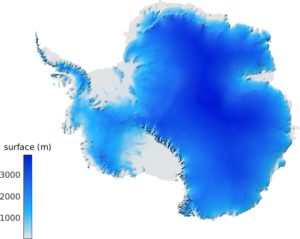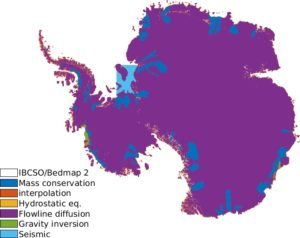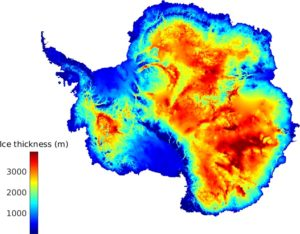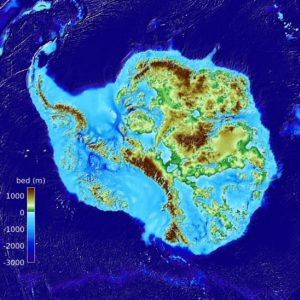# BedMachine Antarctica v2

Since 2014, we have been working on a self-consistent dataset of the Antarctic Ice Sheet based on the conservation of mass that is now freely available at NSIDC.

Note: keep in mind that not all of the bed is mapped using mass conservation (MC). If there is a fast flowing region that is currently not mapped with MC, let me know and I will try to add it in the next release. If you have ice thickness data that is not included, I would also be more than happy to add it to the mapping to further improve the topography.

## Description

The data are in one single file in NetCDF format (795 Mb) and all heights are in meters above mean sea level (the geoid used is provided in the NetCDF file). All the data use the same 450 m-resolution grid although the “true” resolution of the bedrock may vary depending on the method used to map the bed. This dataset uses data from 1993 to 2016 and has a nominal date of 2012 (same as REMA).Antarctic mask The ice/land masks are from ADD rock outcrop, and the floating ice is derived from InSAR grounding lines (pers. comm.). 0 = ocean, 1 = ice-free land, 2 = grounded ice, 3 = floating ice, 4 = lake VostokSurface elevation The surface dem is from REMA.Source Method used to calculate ice thickness: 0 = none, 1 = REMA/IBCSO, 2 = Mass conservation, 3 = interpolation, 4 = hydrostatic equilibrium, 5 = streamline diffusion, 6 = gravity, 7=seismic, 10+ = bathymetry dataIce thickness The ice thickness is inferred using mass conservation along the peryphery of the ice sheet and ordinary kriging in the interior.Bed topography The bed elevation is calculated by subtracting the ice thickness from the surface elevation data.Error map Ice thickness and bed topography error.

For the hydrostatic equilibrium calculation, we used a density of ice ρice=917 kg/m3, and an ocean water density of ρocean=1027 kg/m3 (following the densities used in Griggs & Bamber 2011 and Chuter & Bamber 2015).

As any model output, there are errors in these maps (there is an estimate included in the dataset). Feedback is more than welcome.

## Citation

Morlighem, M., Rignot, E., Binder, T. et al. Deep glacial troughs and stabilizing ridges unveiled beneath the margins of the Antarctic ice sheet. Nature Geoscience 13, 132–137 (2020)

## Disclaimer

The ice thickness and bed topography are model outputs and are not free of error (especially in regions where ice thickness measurements are sparse). This dataset is a work in progress and we encourage users to send us feedback so that we keep improving it.

## Projection

The projection is Polar Stereographic South (71ºS, 0ºE), which corresponds to ESPG 3031

MATLAB now has an extensive library for NetCDF files. filename = 'BedMachineAntarctica-2019-09-04.nc'; x = ncread(filename,'x'); y = ncread(filename,'y'); bed = ncread(filename,'bed')'; %Do not forget to transpose (MATLAB is column oriented) %Display bed elevation imagesc(x,y,bed); axis xy equal; caxis([-1000 3000]);

## Converting heights to WGS84

All heights are referenced to mean sea level (using the geoid EIGEN-6C4). To convert the heights to heights referenced to the WGS84 ellipsoid, simply add the geoid height:

$$z_{ellipsoid}=z_{geoid} + geoid$$

## Surface height and firn depth correction

All the quantities provided in BedMachine are in ice equivalent. This affects primarily the upper surface of the ice, to which we have subtracted a firn depth correction to account for the presence of air in the firn layer. The ice thickness is also in ice equivalent. To recover the top of the surface dem from RAME in WGS84:

$$z_{REMA}= surface + firn + geoid$$

where “firn” is the firn depth correction, “surface” is the surface height, and “geoid” is the geoid height. All these quantities are provided in the netCDF file.

## Acknowledgements and References

This project is performed at the University of California Irvine under a contract with the National Aeronautics and Space Administration (Sea Level Rise Program #NNX14AN03G and MEaSURES-3) and the National Science Foundation (Thwaites #1739031).

The ice thickness data are from:

The bathymetry data are from:

The surface velocity data used by MC are from:

The surface mass balance is from RACMO 2.3 1 km:

The surface topography map is from:

• REMA